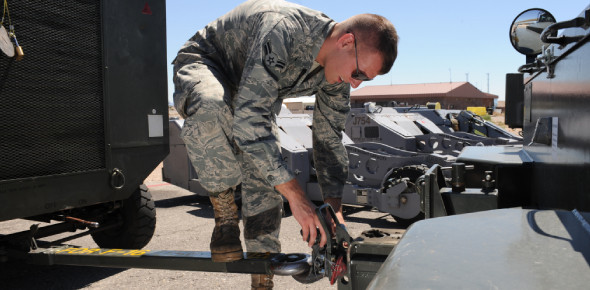# 2A652 CDC Volume 2: Test Questions On Aerospace Ground Equipment!

64 Questions | Attempts: 3846SettingsAerospace ground equipment is responsible for supplying the aircraft with electricity, hydraulic pressure, and air pressure. All equipment must meet requirements for a safe and problem-free flight. With this quiz, you must know how many valence electrons are needed to make a good conductor and what is used to control current flow in a circuit. This quiz explains aerospace ground equipment. Good luck to you.

• 1.
How many valence electrons are needed to make a good conductor?
• A.

3

• B.

4

• C.

5

• D.

6

• 2.
An atom with eight electrons in its outermost shell is said to be
• A.

Free

• B.

Stable

• C.

Valence

• D.

Ionized

• 3.
"Dielectric" is the term used for
• A.

Conductors

• B.

Insulators

• C.

Doped atoms

• D.

Semi-conductors

• 4.
"The flow or drift of electrons through a conductor" in the same direction at the same time is the definition of
• A.

Conductivity

• B.

Voltage

• C.

Current

• D.

Valence

• 5.
The potential difference between two points in a circuit that exerts a force on free electrons is called
• A.

Conductivity

• B.

Voltage

• C.

Current

• D.

Valence

• 6.
What is used to control current flow in a circuit?
• A.

Voltage

• B.

Coulombs

• C.

Resistance

• D.

Conductivity

• 7.
Given a voltage of 24 volts and a resistance of 18 ohms in a series circuit, the current through the resistor would be
• A.

.075 amps

• B.

1.33 amps

• C.

133 amps

• D.

750 amps

• 8.
Total current in a series circuit is equal to the
• A.

Current through one component

• B.

Sum of the current through each component

• C.

Reciprocal of the current through one component

• D.

Reciprocal of the sum of the currents through each component

• 9.
In a parallel circuit, the total voltage is equal to the
• A.

Sum of the voltages across each branch

• B.

Voltage across each resistor

• C.

Voltage across each branch

• D.

The reciprocal of the voltage across the resistance

• 10.
Source current in a parallel circuit will be divided according to branch
• A.

Voltage

• B.

Powers

• C.

Lengths

• D.

Resistances

• 11.
What is the total resistance of a parallel circuit with branch voltages of 440 volt (v) and 440v and branch currents of 4 amp (a) and 16a?
• A.

11 ohms

• B.

22 ohms

• C.

44 ohms

• D.

68.75 ohms

• 12.
Total current in a series-parallel circuit remains the same throughout the
• A.

Parallel portion of the circuit and divides according to component resistance in the series portion

• B.

Series part of the circuit and divides according to branch resistance in the parallel portion

• C.

Parallel portion of the circuit and increases according to component resistance in the series portion

• D.

Series part of the circuit and increases according to component resistance in the parallel portion

• 13.
What is the total current in a series-parallel circuit that has a total voltage of 48 volts (v), a series current of 6 amps (a), one branch current of 2a, and the other branch resistance of 3 ohms?
• A.

3a

• B.

6a

• C.

8a

• D.

12a

• 14.
The area around a magnet where its influence can be felt is the definition of the
• A.

Saturation principle

• B.

Magnetic theory

• C.

Magnetic field

• D.

Law of attraction and repulsion

• 15.
The magnetism that remains after the magnetizing force has been removed is known as
• A.

Electromagnetism

• B.

Molecular magnetism

• C.

Permeability

• D.

Residual magnetism

• 16.
The magnetic field of an electromagnet can be varied by changing the
• A.

Type of core

• B.

Number of turns in the coil

• C.

Amount of current through the coil

• D.

All of the above

• 17.
The electromechanical generation of a voltage requires a magnetic field, relative motion, and
• A.

A complete circuit

• B.

A conductor

• C.

An insulator

• D.

• 18.
In a simple generator, which would be the most difficult method to increase the output?
• A.

Increase the area of the conductor

• B.

Increase the amount of motion

• C.

Increase the strength of the magnetic field

• D.

Increase the relative resistance

• 19.
The principle behind all electromechanical generation is
• A.

Conduction

• B.

Commutation

• C.

Rectification

• D.

Electromagnetic induction

• 20.
An inductor stores energy in the form of
• A.

Heat

• B.

Resistance

• C.

A magnetic field

• D.

An electrostatic field

• 21.
The process by which a current change in one coil produces a voltage in another coil is called
• A.

Inductance

• B.

Self-induction

• C.

Mutual induction

• D.

None of the above

• 22.
A transformer will change all of the following except
• A.

Power

• B.

Current

• C.

Voltage

• D.

Resistance

• 23.
Normally, the winding of a transformer that is connected to a power source is known as the
• A.

Field winding

• B.

Primary winding

• C.

Current winding

• D.

Secondary winding

• 24.
A relay is an electrically operated
• A.

Meter

• B.

Motor

• C.

Switch

• D.

Safety device

• 25.
A relay that once energized, requires a second coil to be energized before the contacts will return to their original positions is called a
• A.

Locking relay

• B.

Latching relay

• C.

Timing relay

• D.

Tripping relay

## Related TopicsBack to top
×

Wait!
Here's an interesting quiz for you.